# 古典密码的演化 （一）— 密码学复习（二）

复习完密码学的基本概念后，下面对古典密码进行简单的复习。下图列出的是经典密码体制的框图。（由于比较懒就直接放笔记的照片了⁄(⁄ ⁄•⁄ω⁄•⁄ ⁄)⁄）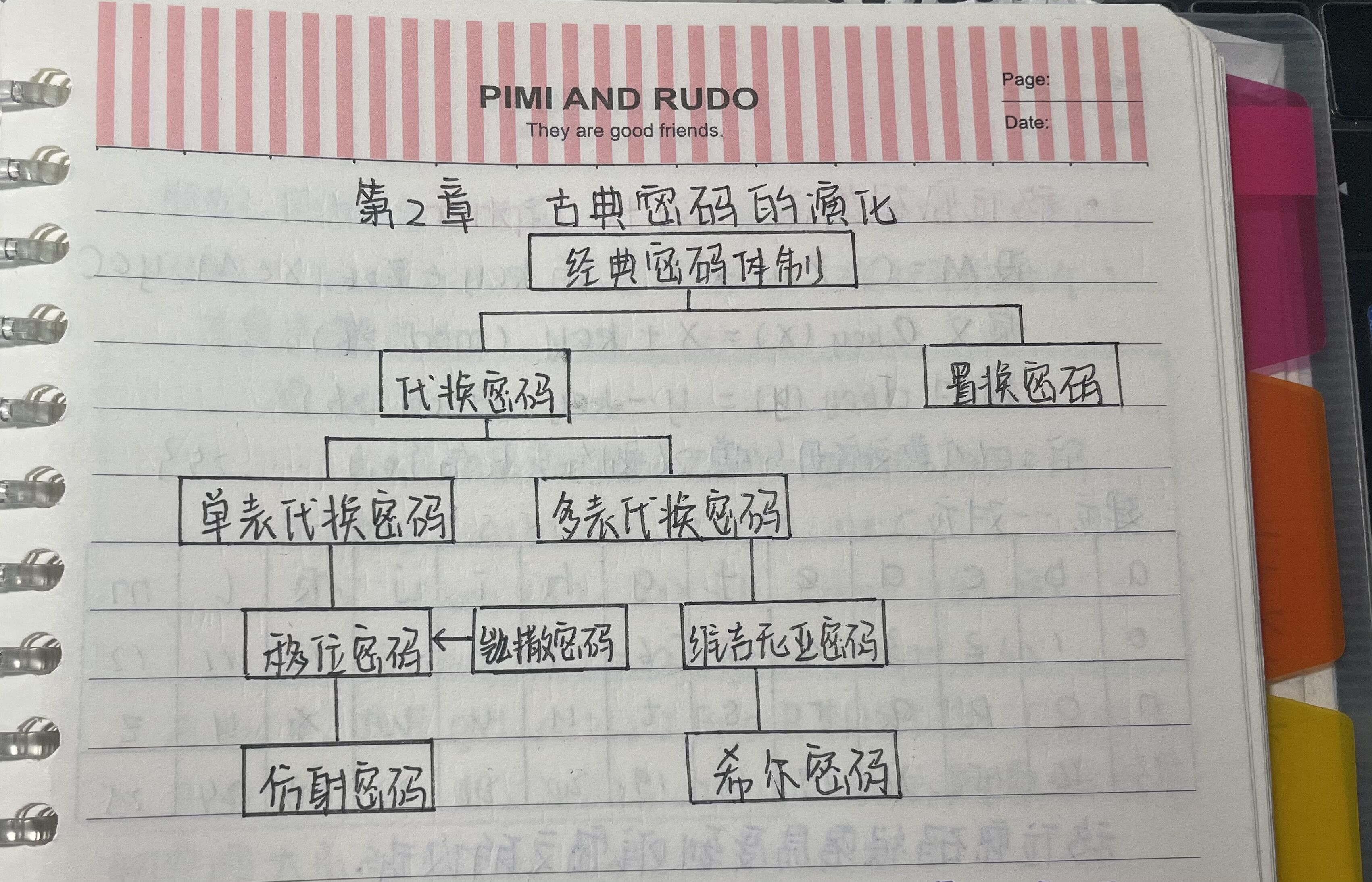古典密码学大体上可以分为 代换密码置换密码

（1）代换密码

构造一个或多个密文字母表，然后用密文字母表中的字母或字母组来代替明文字母或字母组。各字母或字母组的相对位置不变，但其本身改变了。

（2）置换密码

又称换位密码。把明文中的字母重新排序，字母本身不变，但其位置改变了。

### 2.1 移位密码算法 Shift Cipher

设 M = C = Z26,对任意的 key∈Z26, x∈M, y∈C.

定义ekey(x) = x + key (mod 26)

同时dkey(y) = y - key (mod 26)

注：26个英文字母与模26剩余类集合{0,1,...,25}建立一一对应。移位密码很容易受到唯密文的攻击

#### 例子：凯撒密码（key = 3）

若此时明文为venividivici.则密文为：yhqlylglylfl.若解密只需将密文左移三位即可。

### 2.2 仿射密码算法

加密函数取形式为：e(x) = ax + b (mod 26), a,b∈Z26.

要求唯一解的充要条件是gcd(a,26)=1.

注：之所以要求a与26互素。目的是为了保证逆元存在。同时，因为a与26互素，所以a可取φ(26)个。φ(26)=φ(2)φ(13)=1×12=12.故a可取12个。

该算法描述为：

K = {(a,b)∈Z26×Z26; gcd(a,26)=1}

在Z26中，满足gcd(a,26)=1的a只有12个值(1,3,5,7,9,11,15,17,19,21,23,25),因此仿射密码的密钥空间大小为12×26=312个。

对于Z26中与26互素的元素，相应的乘法逆元为：

1-1 mod 26 = 1     3-1 mod 26 = 9     5-1 mod 26 = 21   7-1 mod 26 = 15

9-1 mod 26 = 3    11-1 mod 26 = 19   15-1 mod 26 = 7    17-1 mod 26 = 23

19-1 mod 26 = 11   21-1 mod 26 = 5    23-1 mod 26 = 17   25-1 mod 26 = 25

#### 求逆元过程（以9-1 mod 26 = 3为例）

9·9-1≡1 (mod 26)

9x≡1 (mod 26) 其中x≡9-1 (mod 26)

x = x-1+9-1·26 (mod 26)

= 9-1(1+26)  (mod 26)

= 3       (mod 26)

∴ 9-1≡3 (mod 26)

#### 仿射密码算法例子

设 k=(7,3),注意到7-1 mod 26 = 15,

加密函数：ek(x) = 7x + 3 (mod 26)

解密函数：dk(y) = 15(y-3) (mod 26) = 15x-19 (mod 26)

易见 dk(ek(x)) = dk(7x + 3) = 15(7x + 3)-19 = x+45-19 = x (mod 26)

若加密明文为hot，则有如下步骤：

① 转换字母h、o、t为数字7、14、19

② 然后加密：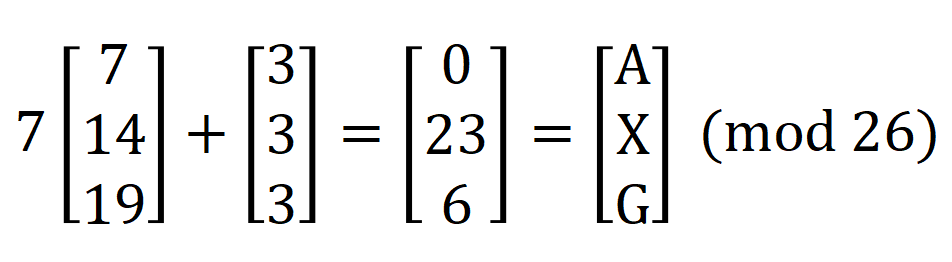③ 解密有：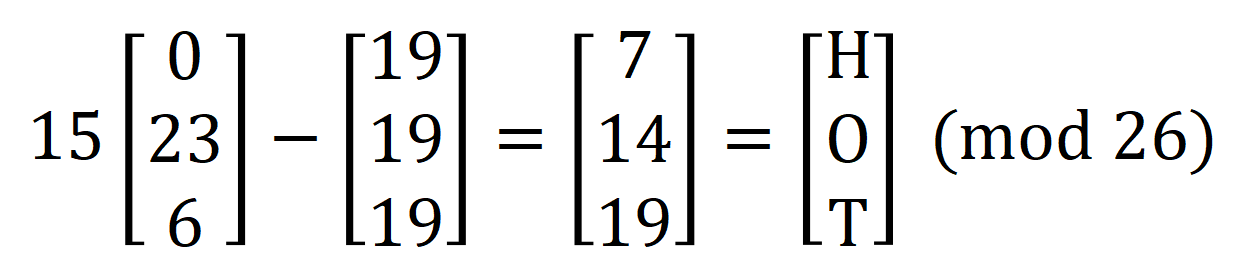### 2.3 单表代换密码 —— 分析

（1）密钥量一般比较小，难以抵抗穷尽搜索攻击

（2）即使密钥量很大，但因为没有将明文字母的频率隐藏起来，所以也很容易受到频数分析法的攻击

### 2.4 单表代换的优缺点

明文字符的形态一般将面目全非。

a.明文的位置不变

b.明文字符相同，则密文字符也相同。从而导致：

① 若明文字符被加密成密文字符a,则明文中e的出现次数就是密文中字符a的出现次数；

② 明文的更随关系反映在密文之中。因此，明文字符的统计规律就完全暴露在密文字符的统计规律之中，形态变但位置不变。

### 2.5 单表代换密码的统计分析举例

密文为：YIFQFMZR...CFWDJNZDIR（共168个字母）

分析：∵Z出现20次，出现的频率约为0.12

∴猜测D(Z)=e

∵出现至少10次的密文字母为C、D、F、J、M、R、Y,出现频率约在0.06到0.095之间

∴猜测{D(C),D(D),D(F),D(J),D(M),D(R),D(Y)}={t,z,o,i,n,s,h,r}...

不太明白的话可以参考

### 2.6 多表代换密码

多表代换密码是一系列（两个以上）代换表依次对明文消息的字母进行代换的方法。可以分为：非周期多表代换密码 和 周期多表代换密码。

非周期多表代换密码：代换表是非周期的无限序列。

周期多表代换密码：代换表个数有限，重复使用。

#### 2.6.1 维吉尼亚密码 Vigenére cipher

设 m 是某固定的正整数，定义P=C=K=(Z26)m,对一个密钥 k=(k1,k2,...,km),定义

ek(x1,x2,...,xm) = (x1+k1,x2+k2,...,xm+km)

dk(y1,y2,...,ym) = (y1-k1,y2-k2,...,ym-km)

且所有的运算都在Z26中。

简单地说，可以将其描述为如下形式：

密钥一般是一个单词或一句话去除后面的重复字母后构成。

本质：多个移位密码

密钥量：对于n为密钥，密钥量有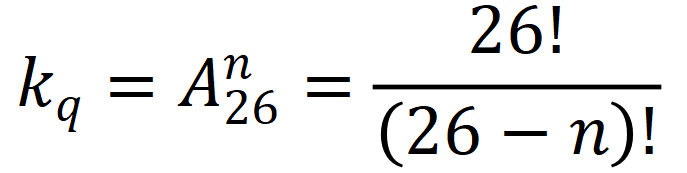例子: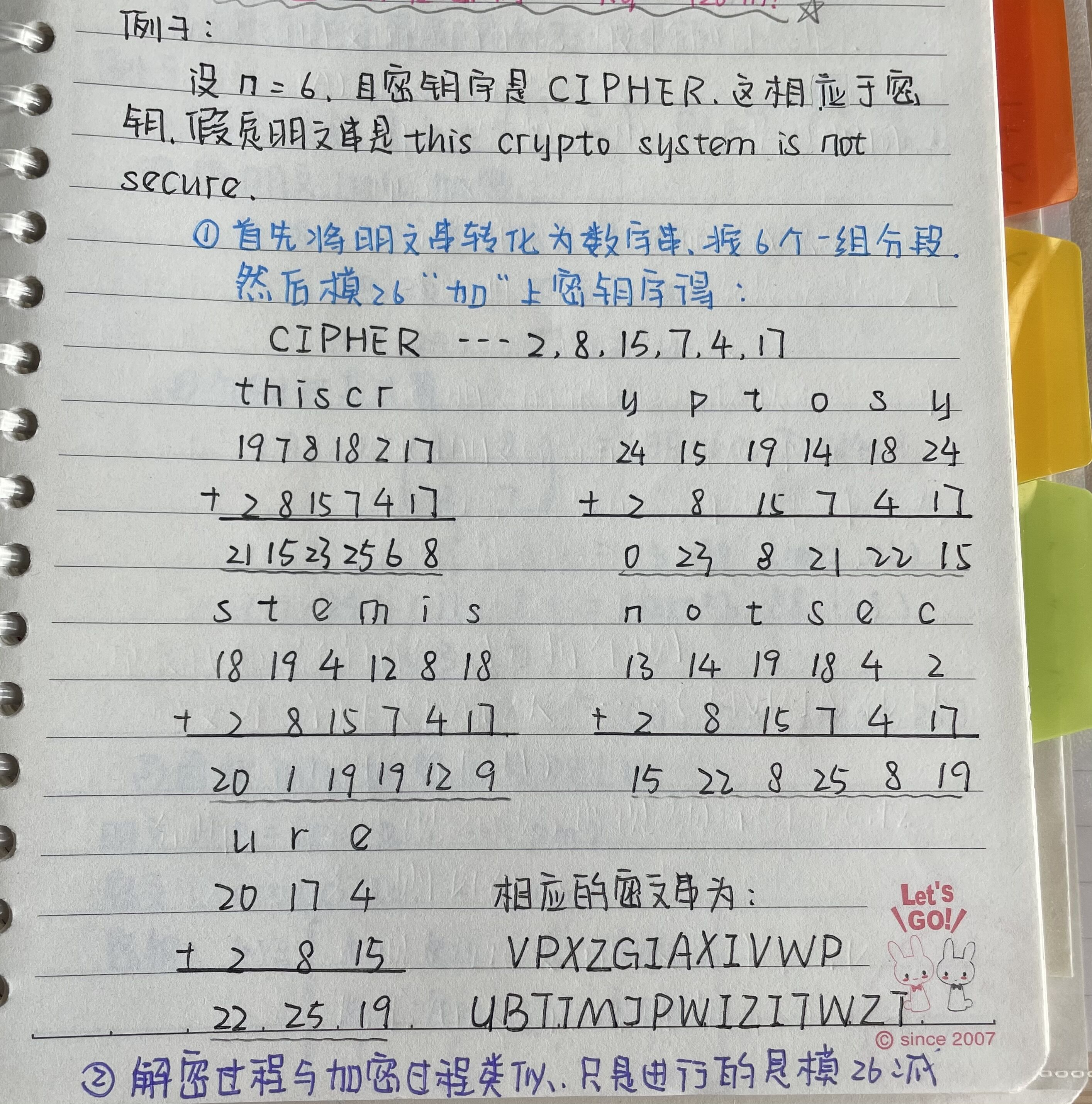#### 2.6.2 希尔密码 Hill cipher

设m是某个固定的正整数，P=C=(Z26)m,又设K={m×m可逆阵，Z26};对任意k∈K,定义

ek(x) = xk

其中所有的运算都是在Z26中进行。

其中明文、密文和密钥可以写成如下形式：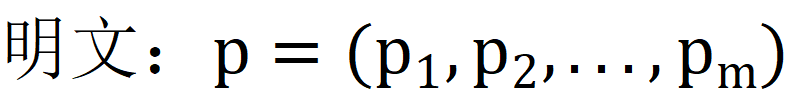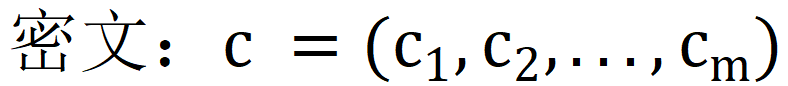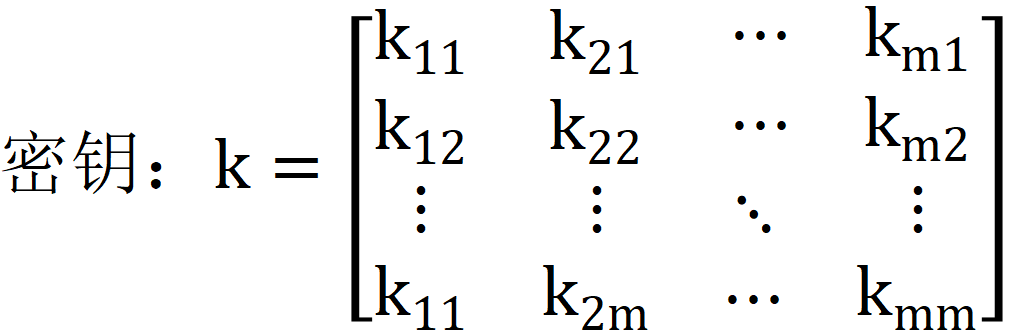注意：

k必须满足条件：

1.非奇异矩阵，即|k|≠0,

2.gcd(|k|,26)=1 (mod 26)

加密：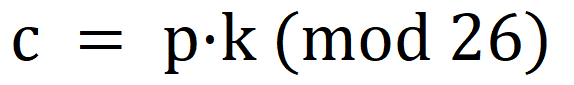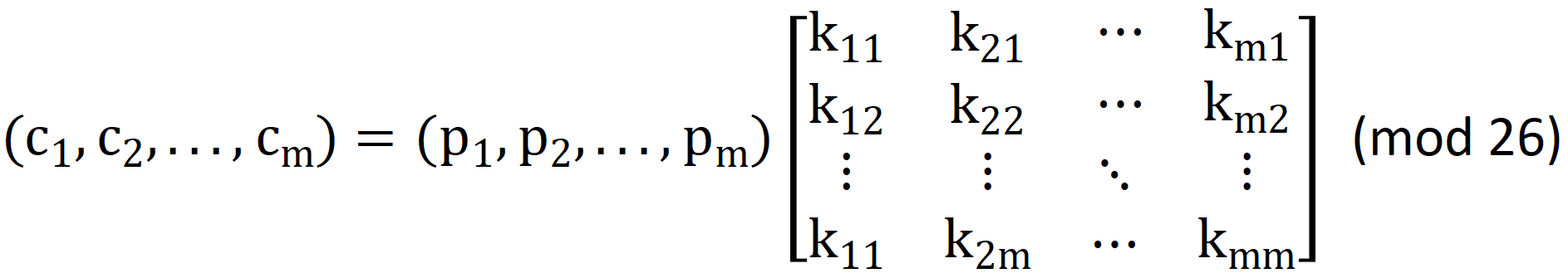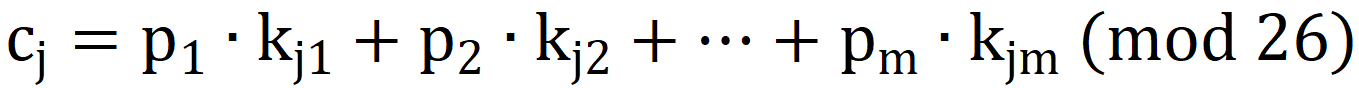解密：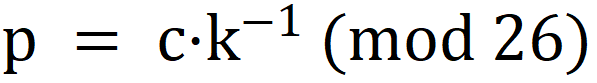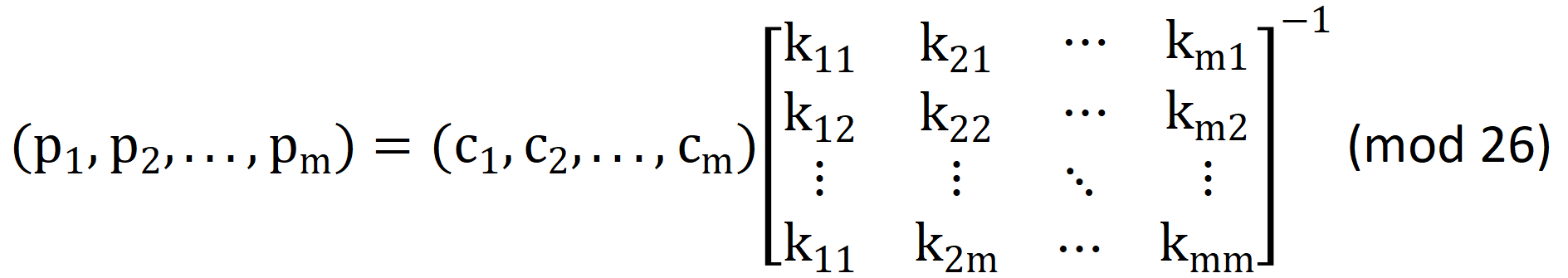例子1：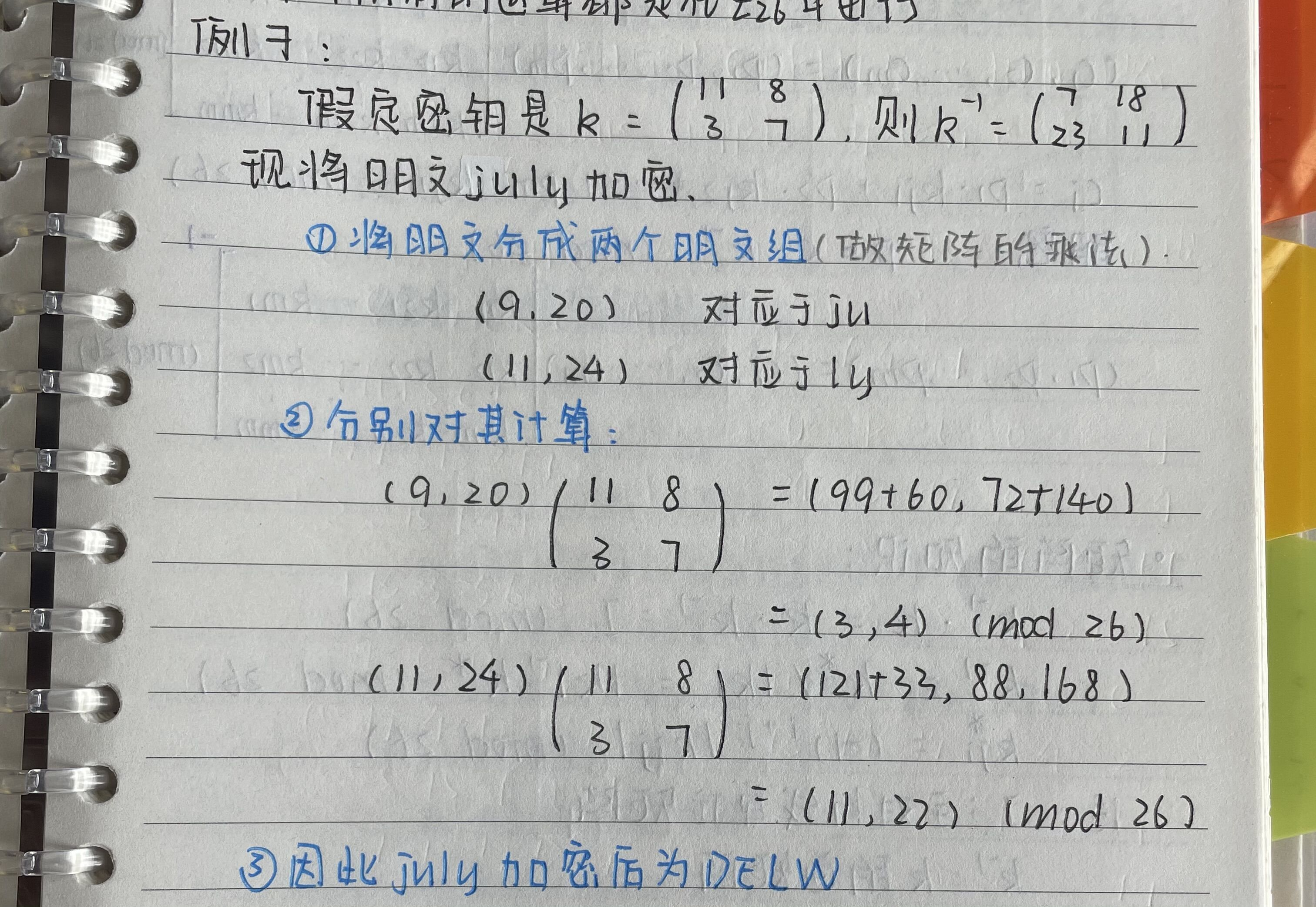例子2：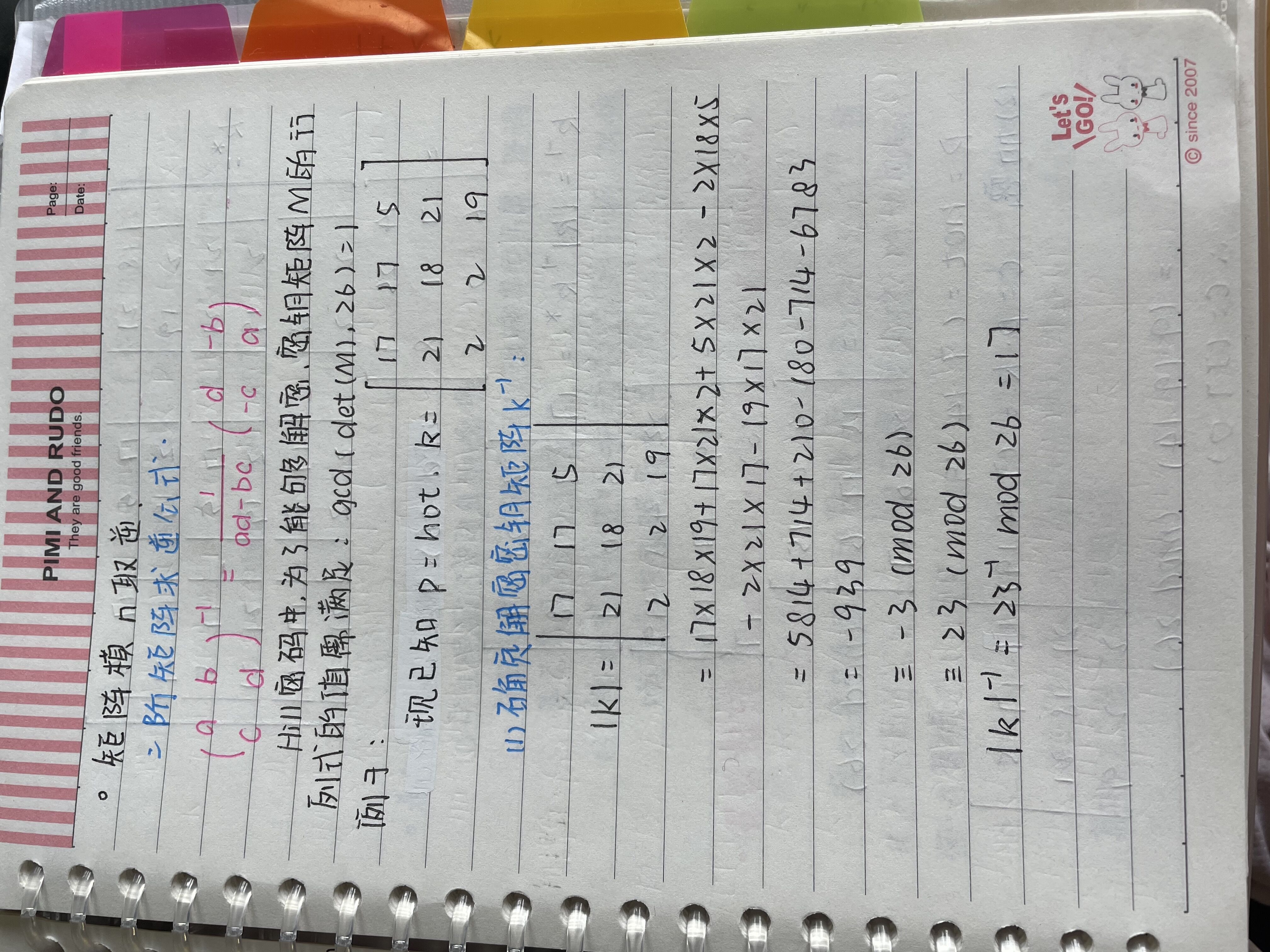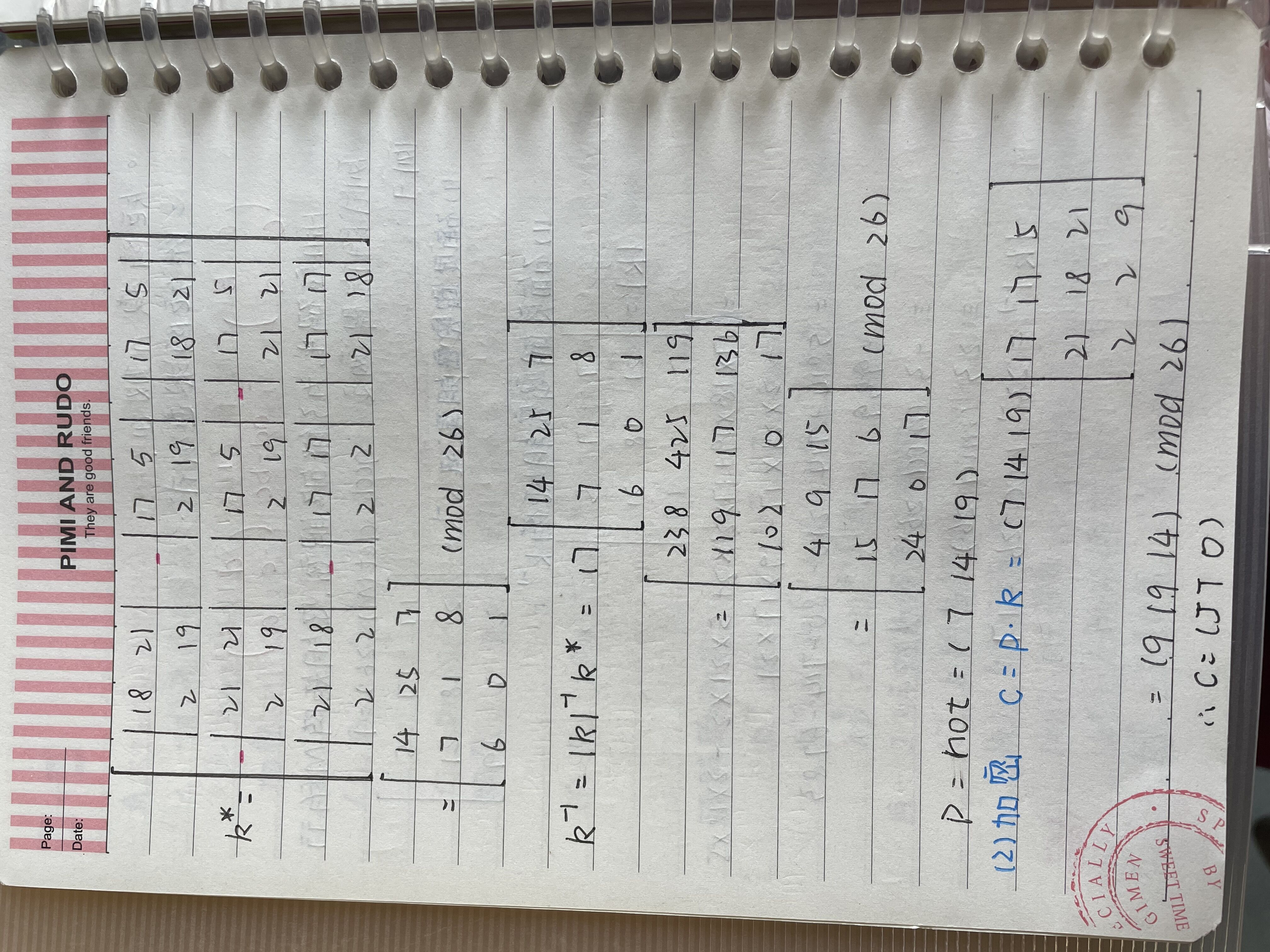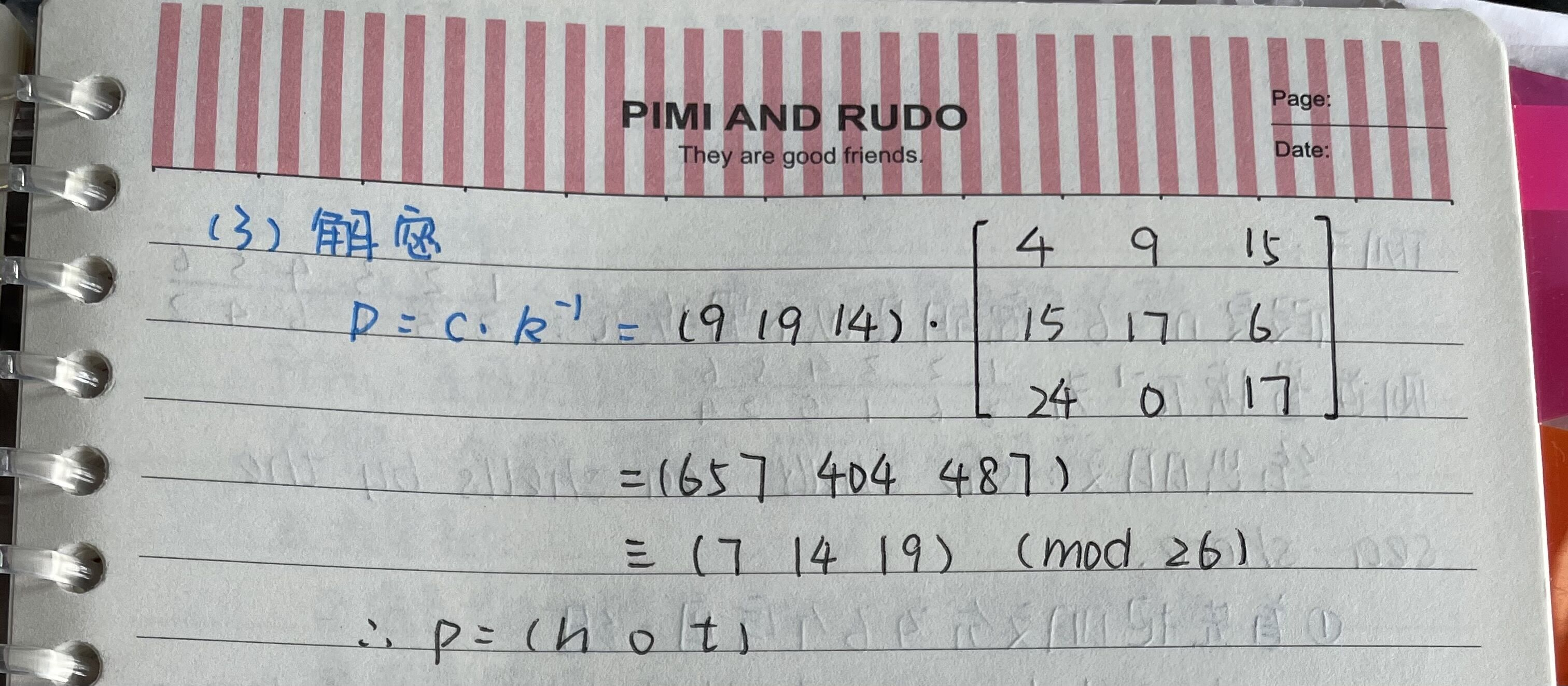介绍完希尔密码后，接下来对希尔密码的特点进行介绍：

（1）完全隐藏了单字母的频数；

（2）字母和数字的对应可以改成其他方案，使得更不容易被攻击成功；

（3）能比较好地抵抗频数法的分析，对抗唯密文攻击强度较高。

### 2.7 置换密码 Permutation Cipher 或 Transposition Cipher

设m是某个固定的正整数，P=C=(Z26)m,且K由所有{1,2,...,m}的置换组成，对一个密钥（即一个置换∏），定义

e(x1,x2,...,xm) = (x∏(1),x∏(2),...,x∏(m))

d(y1,y2,...,ym) = (y-1(1),y-1(2),...,y-1(m))

其中∏-1是∏的逆置换。

例子：在简单对几种古典密码算法进行介绍后，下一篇将介绍密码破译

posted @ 2021-08-01 11:28  不爱学习的Shirley  阅读(941)  评论(0编辑  收藏  举报